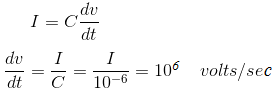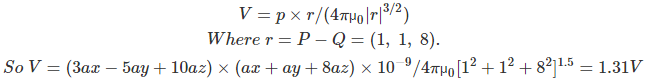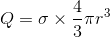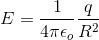# MCQs on Electromagnetic Fields

##### Page 11 of 20. Go to page 1 2 3 4 5 6 7 8 9 10 11 12 13 14 15 16 17 18 19 20
01․ If in a 1 mF capacitor, an instantaneous displacement current of 1 A is to be established in the spaces between its plates then it is possible by applying
106 Amp.
106 Amp/sec.
106 volts.
106 volts/sec.02․ The work done by a force F = 4un - 3uy + 2uz N in giving a 1nC charge a displacement of (10 ux + 2uy - 7uz) m is
20 nj.
40 nj.
60 nj.
100 nj.

Work done = qF.dl = 1(40-6-14) = 20 nj.

03․ A dipole having a moment p – 3ux - 5uy + 10uz ncm is located at p(1,2,-4) in free space. The V as Q(2,3,4) is
1.26 V.
1.31 V.
2.52 V.
2.62 V.

We use the general expression for the potential in the far field:04․ Which statement does not say that electrostatic field is conservative?
If the curl of E is identically zero.
The potential difference between two points is zero.
If is gradient of a scalar potential.
The work done in a closed path inside the fields is zero.

For conversation field curl of E is equal to zero. So, E = - divergence of V and curl of ( – divergence of V) = 0.

05․ A circular ring is carrying uniformly distributed charge q and a point charge – Q on the axis of the ring. The magnitude of dipole moment of the charge system is (Assume distance between centre of ring and point charge is d and radius of ring R)
QR.
QD.
QR2/d.
Q√(R2 + d2).

For points far away, the charge on the ring may be considered to located be at the centre of the ring. Hence the dipolement becomes QD.

06․ If E is the electric field intensity, then what is the value of divergence of (curl of E)?
E.
| E |.
Null vector.
Zero.

We know that divergence of curl of any vector field is zero.

07․ A sphere of 20 cm radius contains electrical charge of density 2/(r sinθ) c/m3. What is the total charge contained within the sphere?
0.335 C.
0.335/sinθ c.
0.
None of above.

Total charge contained within the sphere08․ Two spheres of radius r1 and r2 are connected by a conducting wire. Each of the spheres has been given a charge Q. Now
larger sphere will have greater potential.
larger sphere will have smalled potential.
both of sphere will have same potential.
smaller sphere will have zero potential.

Since both the spheres have same charge. When they are connected by a conducting wire, then the charges flow from higher potential to lower potential and the process stops when they have same potential.

09․ The electrical field strength at a distance point A due to a point charge +q, located at the origin, is 100 µl v/m. If the point charge is now enclosed by a perfectly conducting metal sheet sphere whose centre is at origin, A, outside the sphere, becomes
0.
100 μ v/m.
- 100 μ v/m.
None of above.

The charge +q induces negative charge on the inside of the sphere and positive charge on the outside. If the sphere is enclosed by a Gaussian sphere, the field strength at will be given by Gauss’s law.This the same as through the charge is present at the origin sphere.

10․ Plane z = 10 m carries charge 20 C/m2. The electric field intensity at the origin is
– 10 uz v/m.
– 18 πuz v/m.
– 72 πuz v/m.
– 360 πuz v/m.

The electric field due to intensity plane having charges is E = (ρo/z εo) un Here ρo = 10 - 9/36π F/m. and un is – u (as point below the plane).

<<<910111213>>>